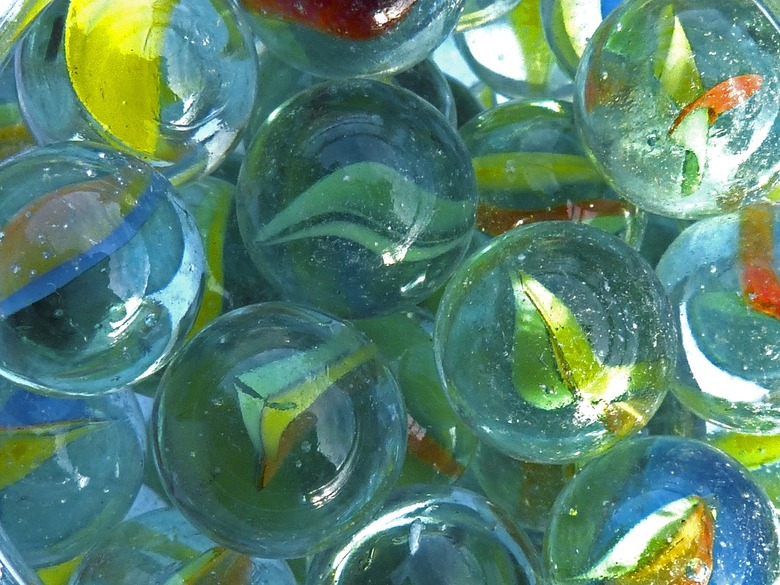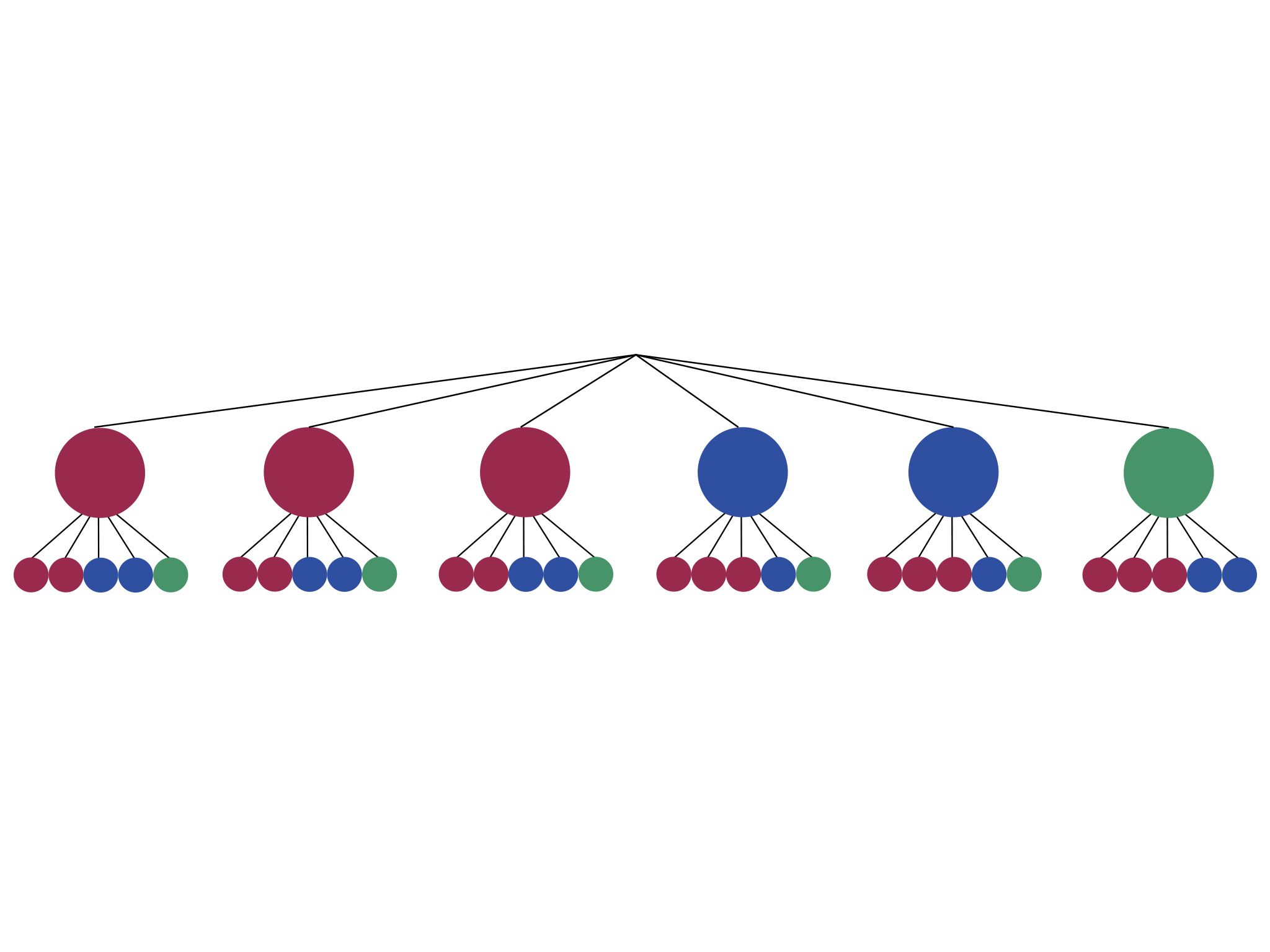Subject:
Statistics and Probability
Material Type:
Lesson Plan
Level:
Middle School
7
Provider:
Pearson
Tags:
• Theoretical Probability
Language:
English
Media Formats:
Text/HTML

# Self Check Exercise## Overview

Students critique and improve their work on the Self Check, then work on additional problems.

# Key Concepts

Students apply what they have learned to date to solve the problems in this lesson.

# Goals and Learning Objectives

• Apply knowledge of probability to solve problems.
• Determine theoretical probability.
• Predict expected results.

# Lesson Guide

Have students review their work and respond to the questions in the Critique. They should work individually for about 10 minutes.

SWD: Students with disabilities may have a more challenging time identifying areas of improvement to target in their assessment. Model explicitly for students (using a student sample) how to review their learning using the intervention questions in order to assess and plan for revisions based on that assessment.

# Critique

Review your work on the Self Check problem and think about these questions:

• Have you found the theoretical probability of each situation (expressed as a ratio)?
• What other ratio is equal to the ratio for theoretical probability?
• How does the number of trials affect how the experimental results compare to the theoretical probability?

# Lesson Guide

Discuss the Math Mission. Students will apply what they know about theoretical and experimental probability.

## Opening

Apply what you know about theoretical and experimental probability.

# Lesson Guide

Organize the class into pairs to revise their work. Encourage students to incorporate ideas from their partner into their work. If several students in the class are struggling with the same issue, you could write a relevant question on the board. You might also ask a student who has performed well on a particular part of the task to help a struggling student.

SWD: Some students with disabilities may struggle with self-assessment; use your knowledge of student strengths and vulnerabilities to inform and create interventions you will put into place for this period of class time.

# Mathematics

While students work in small groups, note:

• Different student approaches to the task
• Students' chosen problem-solving approaches
• Students' choice of math

Attend in particular to the students' mathematical decisions:

• Do they track their progress in the use of their chosen mathematics?
• Do they notice if they have chosen a strategy that does not seem to be productive? If so, what do they do?
• Do students calculate the theoretical probability correctly?
• Do students set up the proportion correctly to find the expected results?
• How do they justify their solution?

Try not to make suggestions that move students toward a particular approach to this task. Instead, ask questions that help students to clarify their thinking.

If students find it difficult to get started, these questions might be useful:

• What do you already know? What do you need to know?
• How can you calculate the theoretical probability?
• How can you compare this to the number of trials to find the expect results?

# Mathematical Practices

Mathematical Practice 1: Make sense of problems and persevere in solving them.

During this lesson, students review their work to make sure that they understand how to solve the problem. They also extend their knowledge by applying it to additional problems.

Mathematical Practice 3: Construct viable arguments and critique the reasoning of others.

In addition to reviewing their own work, students support others and help with their understanding of the concepts.

# Interventions

Student does not calculate the theoretical probability correctly.

• How many marbles are in the bag?
• How many of those are green?
• What is the definition of theoretical probability?

Student does not set up the proportion correctly to find the expected results.

• What does the ratio for the theoretical probability tell you?
• What part of the second ratio is the 200 trials?
• How would you make the number of favorable outcomes out of 200 trials equal to that ratio?

Student does not understand the likelihood of events.

• Are you more likely to get the exact results (or close) with 50 trials or 200?
• Does probability always work out as expected?|

Student provides a poor explanation. For example, the student explains calculations rather than giving mathematical reasons.

• How can you convince a student in another class that your answer is correct?
• Can you find a different way of solving the problem?

1. There are six possible outcomes, but only one is green, so P(green) = $\frac{1}{6}$.
2. Two of the six marbles are blue, so P(blue) = $\frac{2}{6}$ = $\frac{1}{3}$. To find the expected results, a proportion can be set up:
$\begin{array}{ccc}\frac{1}{3}& =& \frac{x}{200}\\ 3x& =& 200\\ x& =& 66.\overline{6}\end{array}$
You could expect to draw a blue marble about 67 times.
3. It is very unlikely that you would draw exactly 67 blue marbles. P(blue) = $\frac{2}{6}$ does not tell you how many times the blue marble will appear, but the likelihood that the blue marble will appear in any given draw.
4. $\frac{2}{6}\cdot 50=16.\overline{6}$. You could expect to draw about 17 blue marbles in 50 trials. It is more likely that the experiment with 200 trials will result in close to 67 blue marbles than the experiment with 50 trials will result in close to 17 marbles. This is because the more trials there are, the closer the results get to the theoretical probability.

## Work Time

Work with your partner to revise your work on the Self Check problem.

Self Check Problem

You have a bag that contains 3 red marbles, 2 blue marbles, and 1 green marble.

1. What is the probability that you will draw a green marble?
2. How many times could you expect to draw a blue marble in 200 trials, based on theoretical probability?
3. What is the likelihood that you would get this exact amount?
4. How would the results for 200 trials compare to results for 50 trials?

# Lesson Guide

Have students extend their work by finding the probability for the new situation.

• P(green, then blue) = $\frac{2}{36}=\frac{1}{18}$
Sample space diagrams will vary. Possible diagram:• P(green, then blue) = $\frac{2}{30}=\frac{1}{15}$
Sample space diagrams will vary. Possible diagram:## Work Time

You have a bag that contains 3 red marbles, 2 blue marbles, and 1 green marble.

• What is the probability that you will draw a green marble and then, after replacing the marble, draw a blue marble? Support your answer with a diagram of the sample space.
• What is the probability that you will draw a green marble and then a blue marble (without replacing the green marble)? Support your answer with a diagram of the sample space.

# Lesson Guide

Organize a whole class discussion to consider issues arising from student's revisions. You may not have time to address all these issues, so focus the discussion on the issues most important for your students.

• Have students share their work and talk about how they approached the problems.
• Have students who had strategies that didn't work share so they can talk about how and when they realized their strategy didn't work and what they did about it.
• Have students share the questions that they addressed and how they addressed those questions.
• Have students ask questions and make observations as they view each other's work.

• What part of the task was most difficult?
• Did your group ever disagree on a solution? Did you resolve your difference of opinion? How?
• ELL:Asking students to reflect on their learning provides opportunities for ELLs to develop literacy in English and proficiency in mathematics. Make sure students use both academic and specialized mathematical language when reflecting on their learning at the end of each session.

# Ways of Thinking: Make Connections

Take notes about your classmates' approaches to revising the Self Check problem and applying their knowledge to a new problem.

• What part of the Self Check problem was most difficult?
• What errors in your work did you find that required revising? How did you go about revising the mistakes?
• Did you and your partner ever disagree about some aspect of the Self Check problem? If so, were you able to resolve your difference of opinion? How?
• How did you use what you learned in the Self Check problem to solve the new problem?